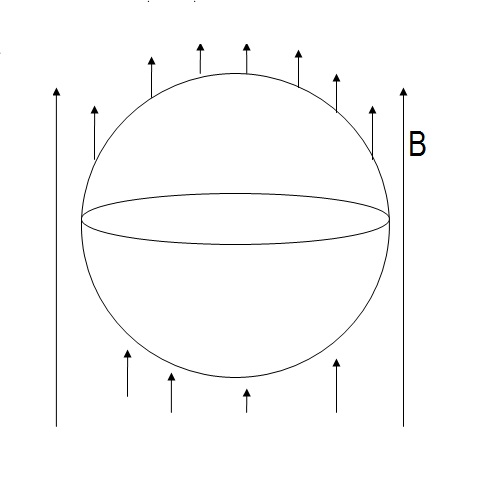# It happens!!

In the arrangement shown, a gas is filled inside a balloon, which is placed in a vertical magnetic field of intensity $B$. The initial volume of balloon is $V_{o}$ and the gas is filled inside it at the rate of $a~m^{3}/s$. If there is no leakage, the emf induced (in mV) at $t=\frac{\pi}{8}~sec$, in a conducting ring which is elastic and placed horizontally along the circumference of balloon is $n$.

[Take $B=1.5~T,~V_{o}=(20 \pi)m^{3},~a=2$]

$\large{Find~n}$×